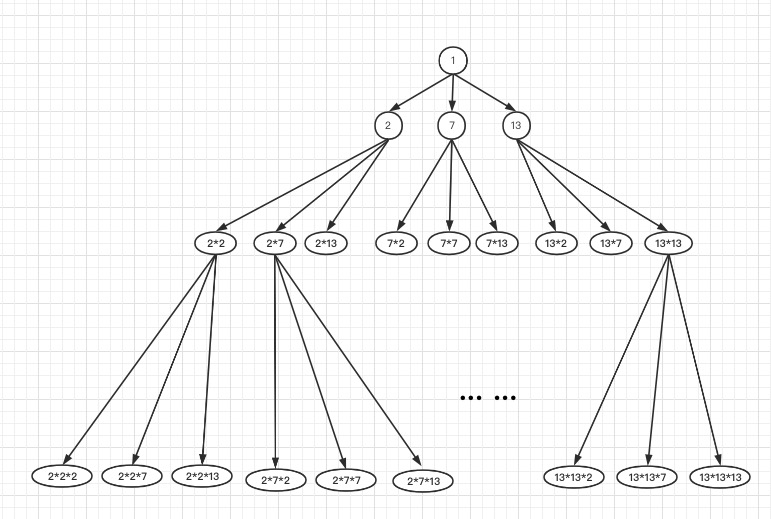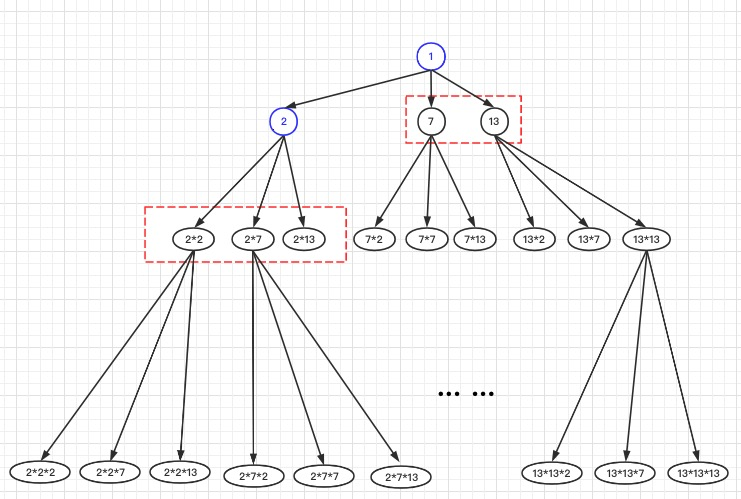Fork me on GitHub

# 算法题 LEECODE 313. 超级丑数

版权声明 本站原创文章 由 萌叔 发表



### 题目

#### 示例

输入: n = 12, primes = [2,7,13,19]


说明:

1 是任何给定 primes 的超级丑数。
给定 primes 中的数字以升序排列。
0 < k ≤ 100, 0 < n ≤ 106, 0 < primes[i] < 1000 。



### 解题思路2• 解空间是一颗多叉数
• 从根沿着树干节点向下，节点的数值越来越大
• 每个节点的孩子节点，都是它的值乘以primes 中的每个数
• 当前的最小值，可能在多个子树中产生2*22*72*1378，下一个最小的超级丑数，只能从这几个数产生。因为剩下的节点都是以上节点的子孙节点，所以肯定要比这些节点中最小值还要大。

### 思路确定

f(n) = min(f(n -1)的子节点, 其它子树的根)


### 代码如下

package main

import (
"container/heap"
"fmt"
)

// An IntHeap is a min-heap of ints.
type IntHeap []int

func (h IntHeap) Len() int           { return len(h) }
func (h IntHeap) Less(i, j int) bool { return h[i] < h[j] }
func (h IntHeap) Swap(i, j int)      { h[i], h[j] = h[j], h[i] }

func (h *IntHeap) Push(x interface{}) {
// Push and Pop use pointer receivers because they modify the slice's length,
// not just its contents.
*h = append(*h, x.(int))
}

func (h *IntHeap) Pop() interface{} {
old := *h
n := len(old)
x := old[n-1]
*h = old[0 : n-1]
return x
}

func nthSuperUglyNumber(n int, primes []int) int {
h := IntHeap([]int{})
heap.Push(&h, 1)
for _, value := range primes {
heap.Push(&h, value)
}
idx := 0
last := 0
var t int
for len(h) > 0 && idx < n {
t = heap.Pop(&h).(int)
//  解决冗余数据
for t <= last {
t = heap.Pop(&h).(int)
}
last = t
for _, v := range primes {
heap.Push(&h, v*last)
}
idx++
}
return last
}

func main() {
n := 550
primes := []int{3, 7, 17, 19, 23, 29, 31, 37, 43,
47, 59, 67, 71, 73, 83, 97, 103, 109, 127, 131, 139, 149, 163, 167, 173, 191, 197, 233, 241, 251}
fmt.Println(nthSuperUglyNumber(n, primes))
}


#### 请我喝瓶饮料